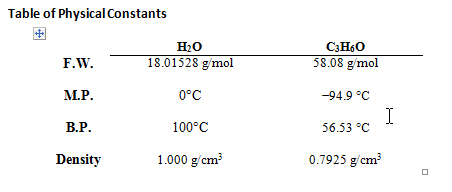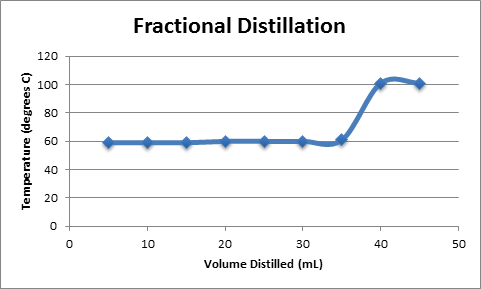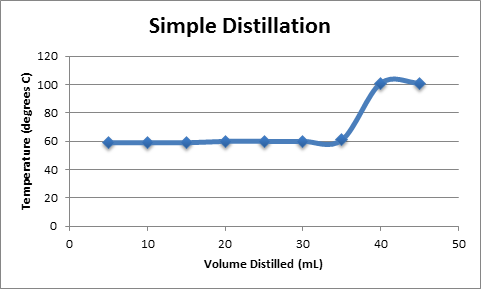# Simple and Fractional Distillation Experiment

Distillation
By: Tim Dimond

Introduction/Purpose
The purpose of this lab was to purify an unknown mixture of acetone/water by both fractional and simple distillation.  This process is a viable method for separation of these two liquids because of the large separation in their boiling points.

Material
-Water and acetone mixture

Equipment
– A setup for fractional distillation and simple distillation
-Volume measuring equipment
-Heating equipment

Procedure
Obtain 70 mL of the acetone/water solution in a round bottom flask with several boiling chips.  Perform a fractional distillation, recording the temperature with every 5 mL of distillate.  Record the weight of the acetone, water, and remaining fractions.  Do the same procedure again, but with a simple distillation set up without a column condenser.Results and Observations

Boiling point of acetone component = ~60 OC based on results
Boiling point of water component = ~ 100 OC based on results
Fractional Distillation
Mixture Weight= 64.68 g
Fraction 1 – 26.17 g, 36 mL
Fraction 2 – 10.01 g, 10.5 mL
Leftover in round bottom flask – 19.98g, 20 mL
DensityFD= 0.727 g/mL
%Acetone= 127%

Simple Distillation
Mixture Weight= 64.98 g
Fraction 1 – 28.04 g, 35 mL
Fraction 2 – 12.45 g, 13 mL
Leftover in round bottom flask – 22.98 g, 24 mL
DensitySD= 0.801 g/mL
%Acetone= 92%

A plot of Temperature vs. Volume of Distillate is shown in Figure 2.  The plot shows a function with a slope of zero, corresponding to the removal of acetone from the solution, followed by a sharp increase in temperature as water begins to boil off from the solution.  Both fractional and simple distillation plots are shown, although they are nearly identical.Questions

4. The fact that our results say the factional distillation yielded 127% acetone is impossible, but may prove that the fractional distillation is more effective.  Further experiment would be necessary, because the 127% value is clearly based on some sort of measuring error.
5. Industry frequently uses distillation for both batch an continuous processes.  One example if distillation in petroleum refineries.
6. The boiling point of a liquid is the temperature at which the vapor pressure of that liquid exceeds the vapor pressure of the air surrounding it.  When this happens, the liquid boils and a phase change occurs, as the liquid becomes a gas.
7. Heating a closed system can cause a build up of pressure and energy, which may eventually result in an explosion or dangerously fast release of this built up energy.

References

– Lab handout
– Wikipedia

• Share